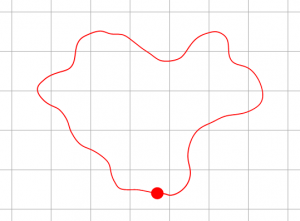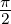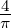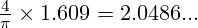## Why hillwalkers should love the Comte de Buffon

Wandering the mountains of the UK has been a big part of my life. You won’t be surprised that before I start a long walk I like to know roughly what I’m letting myself in for. One part of this is estimating how far I’ll be walking.

Several decades ago my fellow student and hillwalking friend David told me of a quick and simple way to estimate the length of a walk. It uses the grid of kilometre squares that is printed on the the Ordnance Survey maps that UK hillwalkers use.

To estimate the length of the walk, count the number of grid lines that the route crosses, and divide by two. This gives you an estimate of the length of the walk in miles.

Yes, miles, even though the grid lines are spaced at kilometre intervals. On the right you can see a made-up example. The route crosses 22 grid lines, so we estimate its length as 11 miles.Is this rule practically useful? Clearly, the longer a walk, the more grid lines the route is likely to cross, so counting grid lines will definitely give us some kind of measure of how long the walk is. But how good is this measure, and why do we get an estimate in miles when the grid lines are a kilometre apart?

I’ve investigated the maths involved, and here are the headlines. They are valid for walks of typical wiggliness; the rule isn’t reliable for a walk that unswervingly follows a single compass bearing.

• On average, the estimated distance is close to the actual distance: in the long run the rule overestimates the lengths of walks by 2.4%.
• There is, of course, some random variation from walk to walk. For walks of about 10 miles, about two-thirds of the time the estimated length of the walk will be within 7% of the actual distance.
• The rule works because 1 mile just happens to be very close tokilometres.

The long-run overestimation of 2.4% is tiny: it amounts to only a quarter-mile in a 10-mile walk. The variability is more serious: about a third of the time the estimate will be more than 7% out. But other imponderables (such as the nature of the ground, or getting lost) will have a bigger effect than this on the effort or time needed to complete a walk, so I don’t think it’s a big deal.

In conclusion, for a rule that is so quick and easy to use, this rule is good enough for me. Which is just as well, because I’ve been using it unquestioningly for the past 35 years.

#### And the Comte de Buffon?

George-Louis Leclerc, Comte de Buffon,  (1707-1788) was a French intellectual with no recorded interest in hillwalking. But he did consider the following problem, known as Buffon’s Needle:

Suppose we have a floor made of parallel strips of wood, each the same width, and we drop a needle onto the floor. What is the probability that the needle will lie across a line between two strips?

Let’s recast that question a little and ask: if the lines are spaced 1 unit apart, and we drop the needle many times, what’s the average number of lines crossed by the dropped needle? It turns out that it iswhere l is the length of the needle. Now add another set of lines at right angles (as if the floor were made of square blocks rather than strips). The average number of lines crossed by the dropped needle doubles toCan you see the connection with the distance-estimating rule? The cracks in the floor become the grid lines, and the needle becomes a segment of the walk. A straight segment of a walk will cross, on average,grid lines per kilometre of its length. Now a mile is 1.609 kilometres, so a segment of the walk will, on average, crossgrid lines per mile, which is very close to 2 grid lines per mile, as our rule assumes. If a mile werekm (1.570… km), we’d average exactly 2 grid lines per mile.

So the fact that using a kilometre grid gives us a close measure of distance in miles is just good luck. It’s because a mile is very close tokilometres.

In the next post, I’ll explore the maths further. We’ll see where the results above come from, and look in more detail at walk-to-walk variability. We’ll also see why results that apply to straight lines also apply to curved lines (like walks), and in doing so discover that not only did the Comte de Buffon have a needle, he also had a noodle.

Mathematical typesetting by QuickLaTeX.